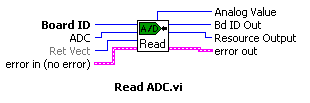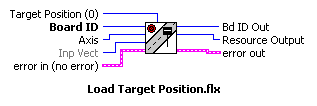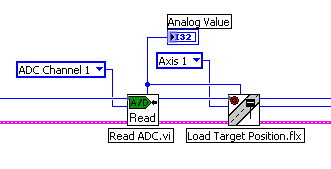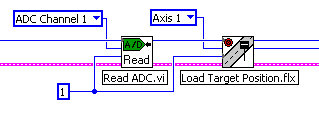# Understanding Input and Return Vectors in Onboard Programming

Publish Date: Sep 06, 2016 | 5 Ratings | 3.60 out of 5 | Print

Many functions that load values and virtually all read back functions support vectoring. Load functions (for example, Load Target Position) take an input vector that specifies the source of the data, either immediate (within the function call itself), from a general-purpose onboard variable, or from an indirect variable. Read functions (for example, Read Position) take a return vector that specifies the destination for the returned data, either the host computer, an onboard variable, or an indirect variable. The ability to use variables in motion control functions is one of the powerful features of the Flexmotion onboard programming environment. You can read data from a resource into a variable, scale or perform some other calculation on the value, and then load the new value as a trajectory or other motion parameter. All data operations functions take data from variables and return the result through a return vector (typically to another variable).

Input and return vectors are very useful when writing onboard programs but have little or no use in programs running on the host computer. For this reason, the default value for input vector is immediate (0xFF) and the API Chapter 4 Software Overview of the Flexmotion Software Reference Manual includes a second “_rtn” version for all Read functions. This version automatically retrieves the data from the RDB after requesting it and returns it by reference to the output parameter. In the case of LabVIEW, you only have one function, and if you want to use the program in the host computer you use the data terminals and wire as you will in a normal LabVIEW program, in case you were to use onboard programming you will make use of the input or return vector terminals and you will specify here to which variable to place the data into; if you would like to make use of the data in further operations just specify the same variable that has the value in to read it.

Example.
In order to understand ‘when’ to use Input or Return Vectors and the difference in LabVIEW or Onboard programming check this example. Imagine the case in which you have an analog voltage being input to the AIN1 pin, which is the ADC of Axis 1, then the decimal value (12 bit) you want to be translated into a target position for Axis 1, this will work for a system in which the analog voltage is in a 1:1 ratio of a target position, and with the analog value you want to control in which position the motor should be. The normal LabVIEW program will have a direct wire connection between the voltage output of the Read ADC function and the Load Target Position function.

First let’s see the Help picture in which the terminals are shown:

Table 1.As we can see the Read ADC function has the output value called ‘Analog Value’ and the Load Target Position function has the input value called ‘Target Position’, we want this two values being 1:1. Also you can see that since Read ADC ‘returns’ a value it has a ‘Return Vector’ input as well. Load Target Position ‘inputs’ a target position value to the function so it has an ‘Input Vector’ input.

The first situation is when you are using plain LabVIEW code and will run on the computer, you may want to have an indicator to show the value read from the Read ADC function in a front panel, and also wire this value directly into the Load Target Position function to command the motor to move, in a LabVIEW type of programming it will be as follows:Figure 1.

Since it is 1:1 for this example we are not making any changes to the value, Read ADC returns the analog voltage not in a voltage value (e.g. 1.75 V), instead, it returns the voltage value in a range of –2048 to +2047 in bipolar range or 0 to 4096 in a unipolar range, we are then commanding the motor to move to that particular target position. Since in LabVIEW the data is passed in between functions using ‘wires’ you don’t need to specify a space in memory where to store the value and where to read it, it is done automatically for you. But in onboard programming you do need to save the value into a variable and use that variable to retrieve the value. The next picture shows how in onboard programming the same code displayed above would be made.Figure 2.

As you can see, we don’t need the data terminals anymore because we are specifying to the Read ADC function to ‘write’ the analog value data into variable ’0x01’, and we are telling Load Target Position to ‘read’ the target position value from the same variable.

In the case of ‘text-based’ programming, if the code runs in the computer then you specify a variable that you have declared or initialized on your code, and in flex_read_adc you will specify this variable to pass the value to, and then in flex_load_target_pos you will reference to the same variable to read the value from, just like the previous example of onboard programming; however, if you use onboard programming, you won’t specify a variable you created, instead, you will specify an onboard variable (hex). You may specify a name if you make use of the #define directive in case of C programming, like shown below:

#define ANALOG_VALUE 0x01

This will let you use ANALOG_VALUE, which is more descriptive than 0x01 when referencing to the analog value variable. For more information on this directive subject please consult a C Reference Manual.

There is a special case in which some functions require more than one variable to pass or receive data to the function; these functions are listed in Appendix D, Table D-1, and Page D-1 of the Flexmotion Software Reference Manual. For this type of call you will need to have consecutive variables dedicated for this functions’ parameters. The number of parameters needed, the number of consecutive variables that need to store the information to pass or receive. The order and number in which the parameters are needed is specified in each parameters’ reference, the table will show depending on the function how many variables are required, and what is the last valid vector you can use, this depends of course, in the number of variables. For example, if we know that the last variable is 0x78, and we need 3 parameters, then last valid variable we can set is 0x76, since parameter number 1 is force to be stored in 0x76, the second parameter in 0x77 and the third parameter in 0x78, you will need to pass to the function the input vector variable holding the first parameter. You are not forced to use the last variables of the list, if you wanted to, you could have used variables 0x11, 0x12 and 0x13, and pass 0x11 as the input vector to the imaginary function we are explaining. The purpose of the table is to show which is the last possible variable you can use as your first parameters’ variable, and pass this as the vector (input/return).

### Ratings

Rate this document

Answered Your Question?
Yes No